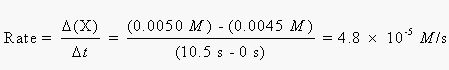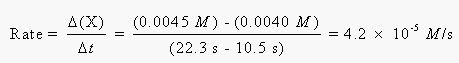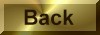Practice Problem 1

Use the data in the above table to calculate the rate at which phenolphthalein reacts with the OH- ion during each of the following periods:

(a) During the first time interval, when the phenolphthalein concentration falls from 0.0050 M to 0.0045 M.

(b) During the second interval, when the concentration falls from 0.0045 M to 0.0040 M.

(c) During the third interval, when the concentration falls from 0.0040 M to 0.0035 M.

Solution

The rate of reaction is equal to the change in the phenolphthalein concentration divided by the length of time over which this cahnge occurs.

(a) During the first time period, the rate of the reaction is 4.8 x 10-5 moles per liter per second:(b) During the second time period, the rate of reaction is slightly smaller:(c) During the third time period, the rate of reaction is even smaller: